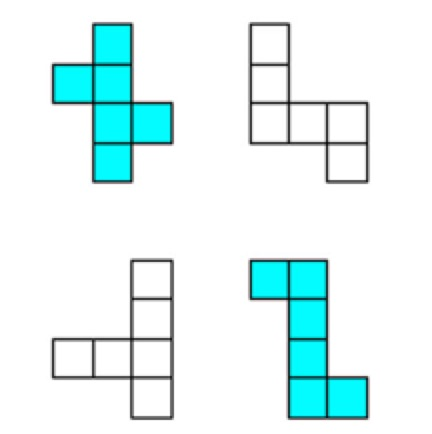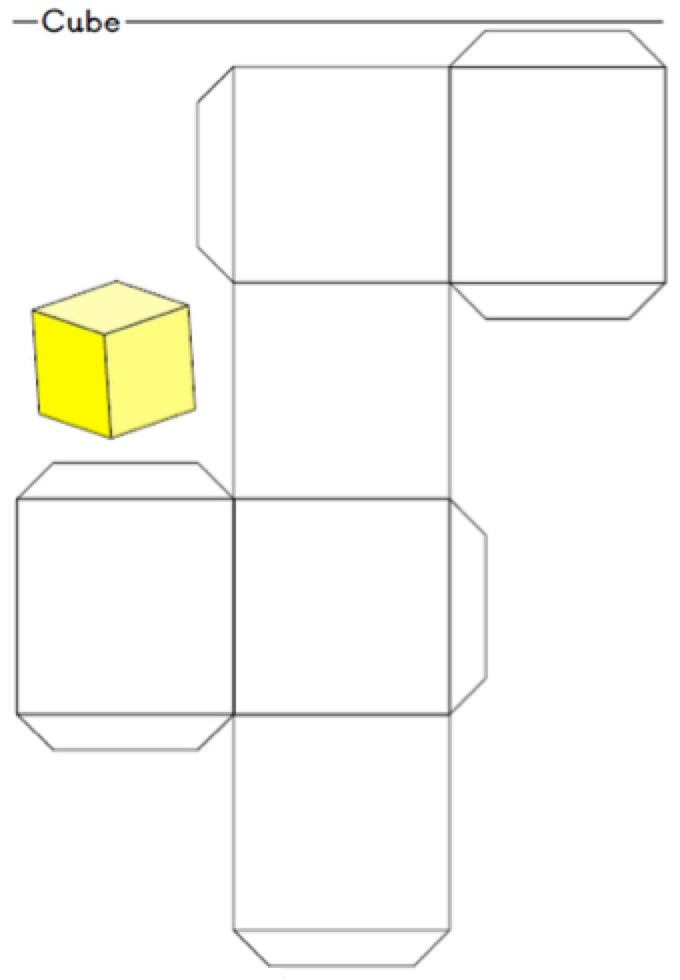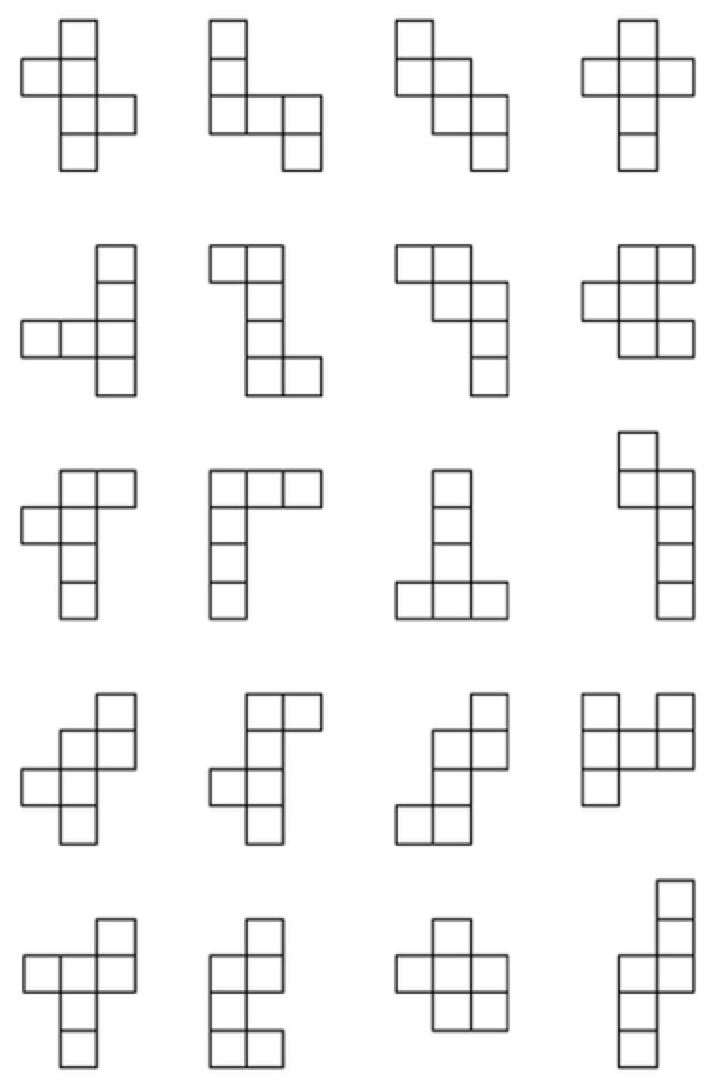Some arrangements of six squares form the net of a cube, others do not. Explain why the two shaded arrangements are nets of cubes and the two unshaded arrangements are not.

Draw thisnet and make your own cube.

How many faces, edges and vertices does a cube have?

Explain how you know that the cube is a regular polyhedron.

Which of these arrangements ofsix squares make a net for a cube and which do not?

What can you say about the symmetries of the cube? Describe all the planes of symmetry. Describe all the axes of rotational symmetry and give the order of rotational symmetry for each axis.